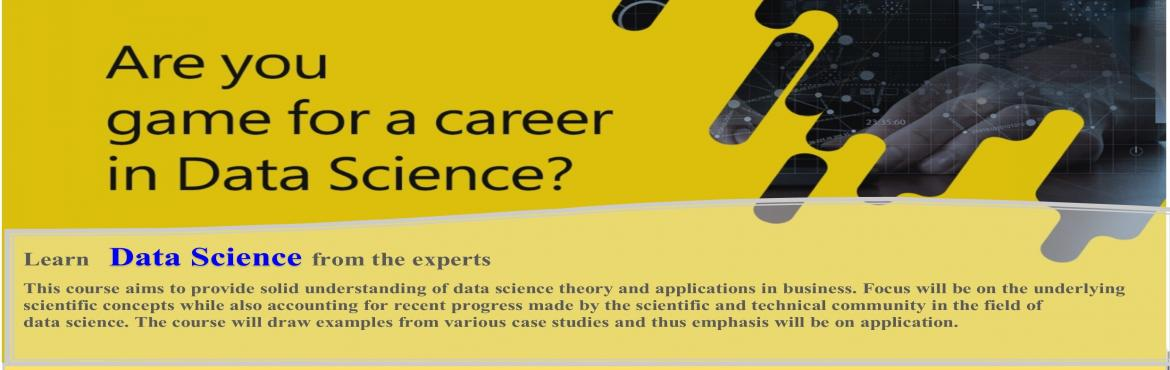# Applied Machine Learning and Data Analytics

• ## Special Offer

Sale Date Ended

INR 25000
Sold Out
• ## Early Bird Offer

Sale Date Ended

INR 30000
Sold Out
• ## Entry Pass

Sale Date Ended

INR 35000
Sold Out

#### Invite friends

Page Views : 78

Course Duration:              80 Hours

Course Evaluation:           Module wise quizzes and a projects

Course Objective:             This course aims to provide solid understanding of data science theory and applications in business. Focus will be on the underlying scientific concepts while also accounting for recent progress made by the scientific and technical community in the field of data science. The course will draw examples from various case studies and thus emphasis will be on application. Associate shall get a hands-on experience in the application of these techniques for addressing practical problems from retail domain.

Instructor's Bio:

The course will be conducted by an Assistant Professor at IIT Kharagpur. His teaching interests include non-linear programming, multivariate statistical models, generalized linear models, machine learning and data analytics. He has offered various courses, lectures and workshops on applied machine learning and data analytics at IIT Kharagpur and other premier institute.

Course Content

Module A: Basics (20 Hours)

A.1         Probability and Statistics basics

1. Sample Space and Events
2. Random variables
3. Sampling and distributions
4. Parameter Estimation
5. Hypothesis Testing
6. Multivariate Normal Distribution (Gaussian)
7. ANOVA, ANCOVA, MANOVA, MANCOVA
8. Non-parametric Tests

A.2         Matrix Algebra and Random Vectors basics

1. Matrix and Vector Algebra
2. Positive Definite Matrices
3. Square Root Matrix
4. Random Vectors and Matrices
5. Matrix Inequalities and Maximization
6. Eigen Values and Eigen vectors
7. Spectral Decomposition
8. Singular Value Decomposition (SVD)
9. Non Negative Matrix Factorization (NMF)

A.3         Multivariable Calculus and optimization basics

1. Multivariate Differential & Integral calculus
2. Directional Derivatives
3. Convex/Non-convex functions
4. Fermat’s Theorem
5. Lagrange function
6. KKT conditions
7. Multivariable Search techniques

i.     Derivative-free: Dichotomous,  Fibonacci, Golden selection

ii.     Derivative based: Steepest Descent, Gradient descent, Newton’s method

iii.     Random Search: Genetic Algorithm

A.4         Python Programming basics

Module B: Learning Concepts (10 Hours)

1. Supervised and Unsupervised Learning
3. Model Selection
4. Cross Validation
5. VC Dimension
6. Regularization theory

Module C: Statistical Learning Models (20 Hours)

1. Multivariate Linear regression
2. Principle and Factor Analysis
3. Discrimination and Classification
4. Multivariate normal
5. Fishers linear discriminant
6. Clustering
7. Expectation Maximization
8. K-means, Hierarchal and DBSCAN
9. Generalized Linear Models (Exponential Family)
10. Logistic and Multinomial regression
11. Poisson regression and log-linear models
12. Survival models

Module D: Machine Learning Models (30 Hours)

1. Artificial Neural Network
2. Deep Learning
3. Support Vector Machines and Kernel Regression
4. Hidden Markov Models
5. Decision Trees
6. Ensemble Methods
7. Random Forest